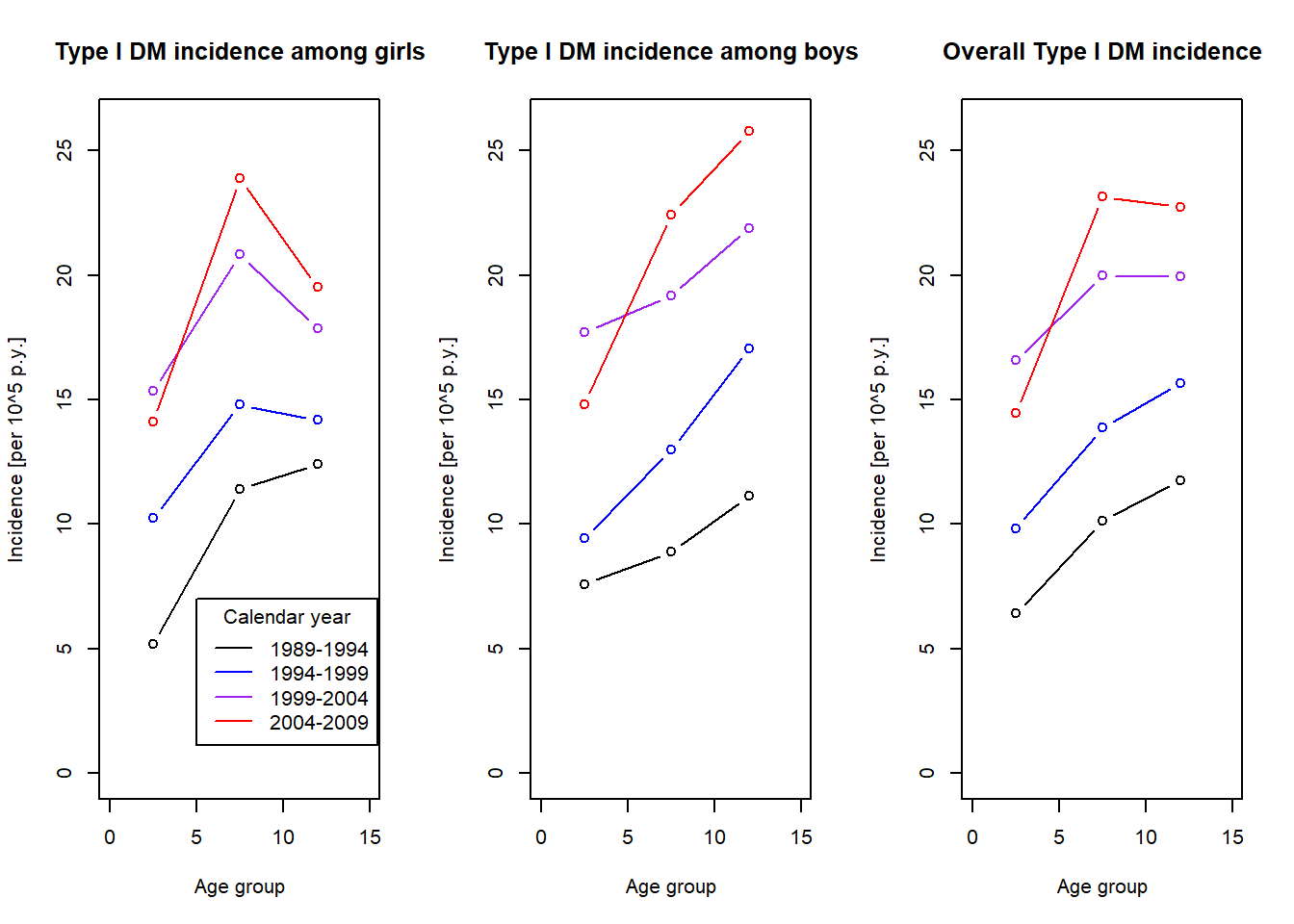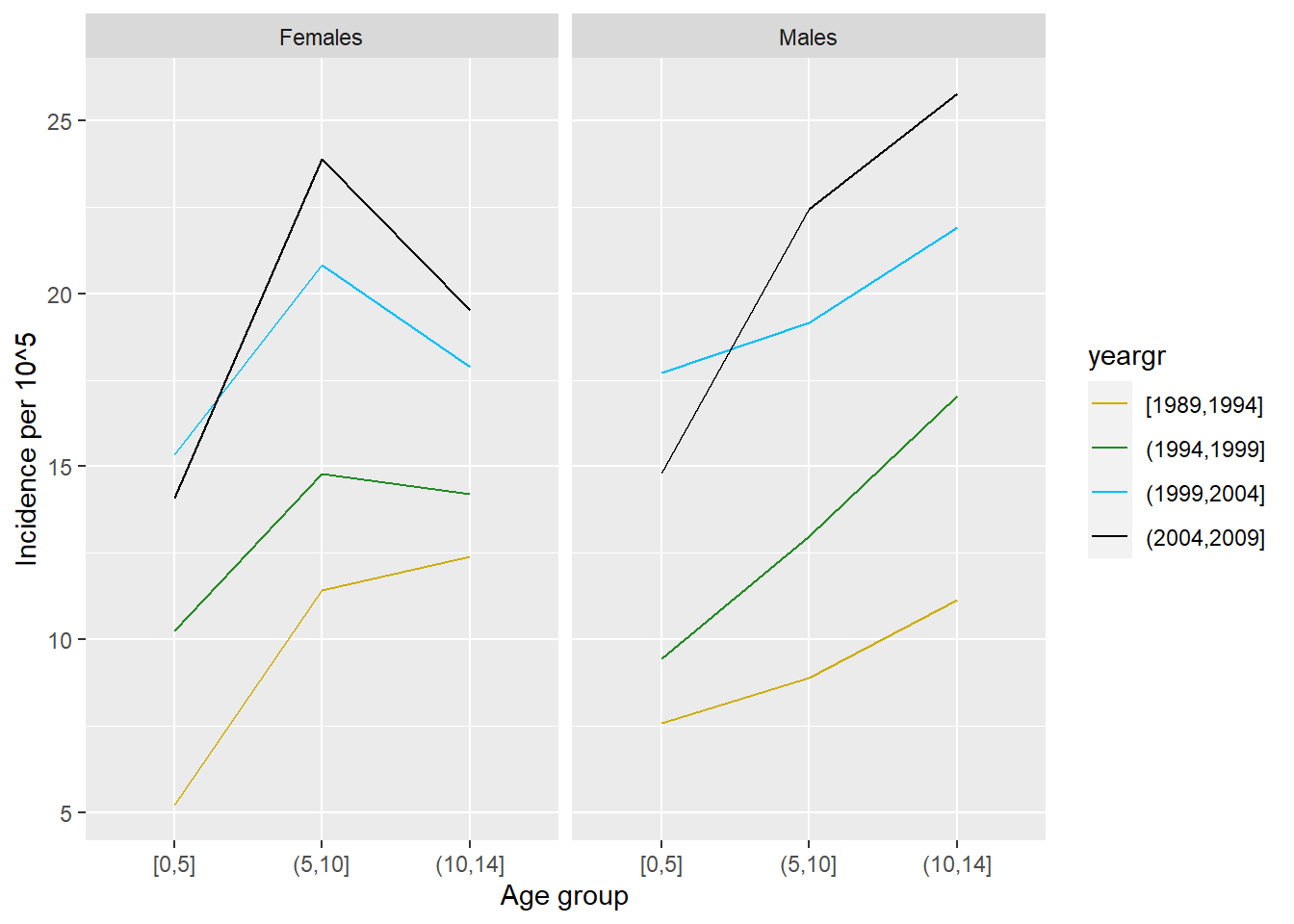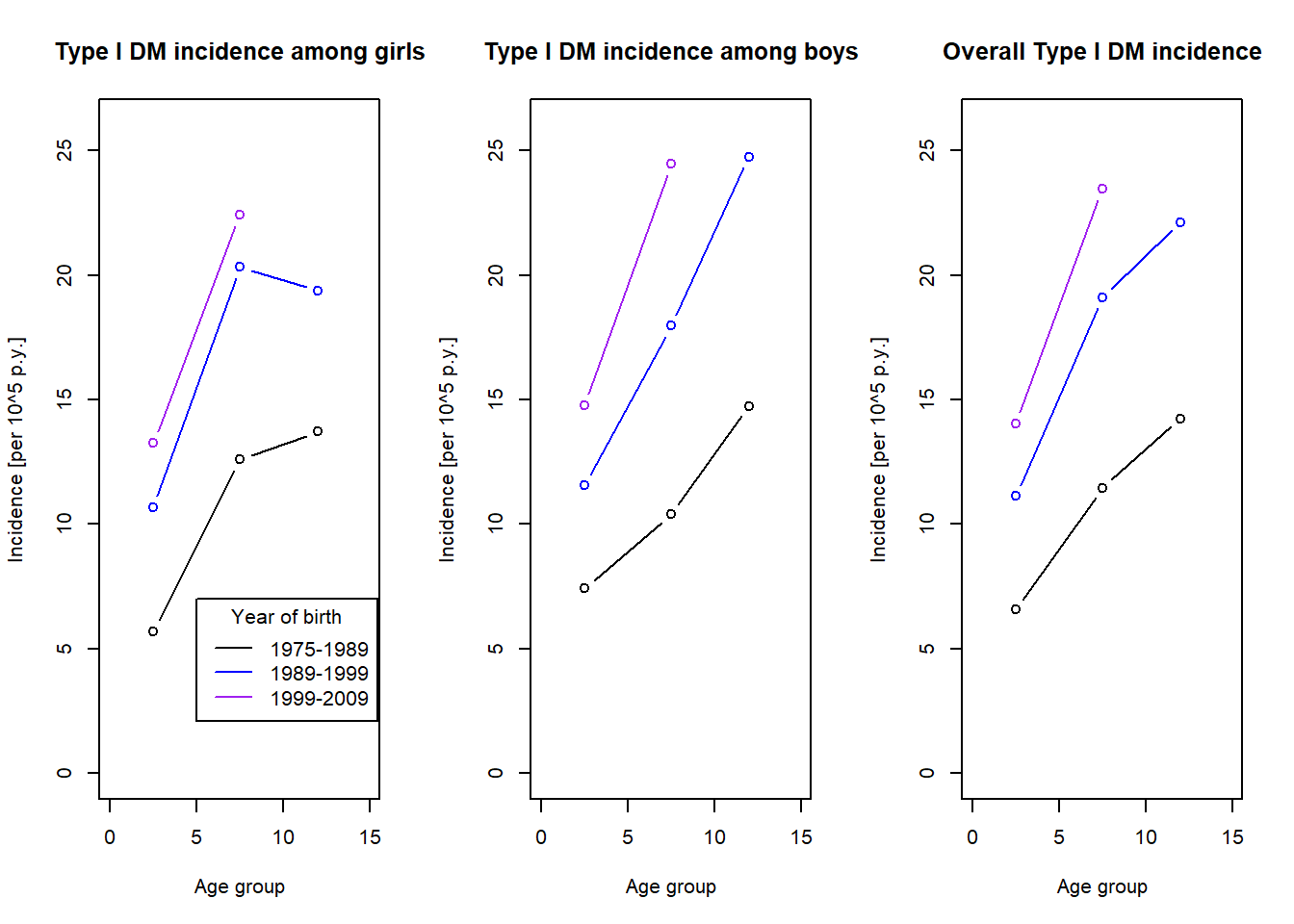# Assignment 1: Estimating incidence rates

Author

Martin Otava

 4
library(dplyr)
library(ggplot2)
library(Epi)

## Assignment

The dataset dbtr (here) contains the numbers of cases of Type 1 Diabetes Mellitus observed in the Czech Republic, together with the population size, aggregated by sex, age (0 to 14) and calendar year (1989-2009).

1. Estimate age-specific incidence rates of Type 1 DM by calendar year for boys, girls and all children. [Decide whether and how age and calendar year should be grouped.] Plot estimated incidence rates against age for (i) different time periods; (ii) different birth cohorts. Do you feel that incidence of Type 1 DM changed between 1989 and 2009?
2. Estimate the cumulative risks (i.e., probabilities) of developing Type 1 DM before the 15th year of age at different time periods (again for boys, girls, and all together).
3. Calculate age-standardized incidence rates for Type 1 DM at different time periods (again for boys, girls, and all together). [Age standardized rates combine age-specific rates over the same standard age distribution at all periods.]
4. Calculate confidence intervals for cumulative risks and age-standardized incidence rates.

## Data

### Classical R framework

First some data exploration

load("dbtr.RData")
dim(dbtr)
 630   5
names(dbtr)
 "year"    "age"     "sex"     "gen.pop" "cases"  
head(dbtr)
  year age     sex gen.pop cases
1 1989   0 Females   62283     0
2 1989   0   Males   65008     0
3 1989   1 Females   64266     1
4 1989   1   Males   67130     0
5 1989   2 Females   63051     4
6 1989   2   Males   66486     6

Next step, we prepare some useful quantities from the raw data set.

## Calculate year of birth
dbtrAnalysis <- dbtr
dbtrAnalysis$byear <- with(dbtrAnalysis, year-age) head(dbtrAnalysis)  year age sex gen.pop cases byear 1 1989 0 Females 62283 0 1989 2 1989 0 Males 65008 0 1989 3 1989 1 Females 64266 1 1988 4 1989 1 Males 67130 0 1988 5 1989 2 Females 63051 4 1987 6 1989 2 Males 66486 6 1987 summary(dbtrAnalysis)  year age sex gen.pop cases Min. :1989 Min. : 0 Females:315 Min. :43037 Min. : 0.000 1st Qu.:1994 1st Qu.: 3 Males :315 1st Qu.:46887 1st Qu.: 5.000 Median :1999 Median : 7 Median :61609 Median : 8.000 Mean :1999 Mean : 7 Mean :58696 Mean : 8.183 3rd Qu.:2004 3rd Qu.:11 3rd Qu.:65809 3rd Qu.:11.000 Max. :2009 Max. :14 Max. :95594 Max. :24.000 byear Min. :1975 1st Qu.:1987 Median :1992 Mean :1992 3rd Qu.:1997 Max. :2009  ## Group calendar year in five-year intervals range(dbtrAnalysis$year)
 1989 2009
#  1989 2009
(yearpts <- seq(1989, 2009, by=5))
 1989 1994 1999 2004 2009
dbtrAnalysis$yeargr <- cut(dbtrAnalysis$year, yearpts, include.lowest = TRUE)
table(dbtrAnalysis$yeargr, useNA = "a")  [1989,1994] (1994,1999] (1999,2004] (2004,2009] <NA> 180 150 150 150 0  ## Group age in five-year intervals range(dbtrAnalysis$age)
  0 14
agepts <- c(0, 5, 10, 14)
dbtrAnalysis$agegr <- cut(dbtrAnalysis$age, agepts, include.lowest = TRUE)
table(dbtrAnalysis$agegr, useNA = "a")  [0,5] (5,10] (10,14] <NA> 252 210 168 0  ## Group yer of birth in ten-year intervals range(dbtrAnalysis$byear)
 1975 2009
birthpts <- c(1975, 1989, 1999, 2009)
dbtrAnalysis$birthgr <- cut(dbtrAnalysis$byear, birthpts, include.lowest = TRUE)
table(dbtrAnalysis$birthgr, useNA = "a")  [1.98e+03,1.99e+03] (1.99e+03,2e+03] (2e+03,2.01e+03] <NA> 240 280 110 0  ### Same data generated in tidyverse We can do the same in tidyverse in a bit more concise way. It is easier to read, but not possible to simply check the steps in between. Also note that we had to epxlore the range of the data aforehand to determine cut-offs, but that can be done easily from summary statement. dbtrAnalysis2 <- dbtr %>% mutate(byear = year-age) %>% mutate(yeargr = cut(year, seq(1989, 2009, by = 5), include.lowest = TRUE)) %>% mutate(agegr = cut(age, c(0,5,10,14), include.lowest = TRUE)) %>% mutate(birthgr = cut(byear, c(1975, 1989, 1999, 2009), include.lowest = TRUE)) head(dbtrAnalysis2)  year age sex gen.pop cases byear yeargr agegr birthgr 1 1989 0 Females 62283 0 1989 [1989,1994] [0,5] [1.98e+03,1.99e+03] 2 1989 0 Males 65008 0 1989 [1989,1994] [0,5] [1.98e+03,1.99e+03] 3 1989 1 Females 64266 1 1988 [1989,1994] [0,5] [1.98e+03,1.99e+03] 4 1989 1 Males 67130 0 1988 [1989,1994] [0,5] [1.98e+03,1.99e+03] 5 1989 2 Females 63051 4 1987 [1989,1994] [0,5] [1.98e+03,1.99e+03] 6 1989 2 Males 66486 6 1987 [1989,1994] [0,5] [1.98e+03,1.99e+03] ## Age-specific incidence: Calculated over aggregated calendar period/birth cohort group ### Age-specific incidence by time period We first need to calculate the number of cases for each category of age-calendar year grouping. Then, we need to get general population for each of these categories. Dividing these two gives us the incidence. (casetbl <- with(dbtrAnalysis, tapply(cases,list(agegr, yeargr, sex), sum))) , , Females [1989,1994] (1994,1999] (1999,2004] (2004,2009] [0,5] 107 145 186 191 (5,10] 229 230 272 264 (10,14] 236 185 223 199 , , Males [1989,1994] (1994,1999] (1999,2004] (2004,2009] [0,5] 164 141 227 212 (5,10] 187 212 264 262 (10,14] 222 233 287 277 # children of age 0 were born only during that year, so they do not contribute same time period as other age categories # hence, we adjust the population at age 0 as workaround dbtrAnalysis$gen.pop0 <- with(dbtrAnalysis, ifelse(age==0, gen.pop/2, gen.pop))
poptbl <- with(dbtrAnalysis, tapply(gen.pop0, list(agegr, yeargr, sex), sum))

(inctbl <- casetbl/poptbl*10^5)
, , Females

[1989,1994] (1994,1999] (1999,2004] (2004,2009]
[0,5]      5.202604    10.25468    15.35798    14.10178
(5,10]    11.420985    14.79652    20.82183    23.89683
(10,14]   12.405031    14.19371    17.86912    19.51832

, , Males

[1989,1994] (1994,1999] (1999,2004] (2004,2009]
[0,5]      7.589460    9.452331    17.71633    14.82430
(5,10]     8.886135   12.989981    19.16641    22.43723
(10,14]   11.132284   17.050436    21.89785    25.76612

Without gender, we can get following:

(inctbl.pop <- apply(casetbl,c(1,2),sum)/apply(poptbl,c(1,2),sum)*10^5)
        [1989,1994] (1994,1999] (1999,2004] (2004,2009]
[0,5]      6.425525    9.842779    16.57037    14.47286
(5,10]    10.122928   13.871249    19.97220    23.14681
(10,14]   11.753674   15.655853    19.93282    22.72499

Now plotting all the results.

par(mfrow=c(1,3))

plot((agepts[-1]+agepts[-4])/2,inctbl[,1,"Females"],xlim=c(0,15),
ylim=c(0,26),type="b",xlab="Age group",ylab="Incidence [per 10^5 p.y.]")
title(main="Type I DM incidence among girls")
lines((agepts[-1]+agepts[-4])/2,inctbl[,2,"Females"],type="b",col="blue")
lines((agepts[-1]+agepts[-4])/2,inctbl[,3,"Females"],type="b",col="purple")
lines((agepts[-1]+agepts[-4])/2,inctbl[,4,"Females"],type="b",col="red")

a <- legend(x=5,y=7,lty=1,col=c("black","blue","purple","red"),
legend=paste(yearpts[-5],yearpts[-1],sep="-"),
title="Calendar year")

plot((agepts[-1]+agepts[-4])/2,inctbl[,1,"Males"],xlim=c(0,15),
ylim=c(0,26),type="b",xlab="Age group",ylab="Incidence [per 10^5 p.y.]")
title(main="Type I DM incidence among boys")
lines((agepts[-1]+agepts[-4])/2,inctbl[,2,"Males"],type="b",col="blue")
lines((agepts[-1]+agepts[-4])/2,inctbl[,3,"Males"],type="b",col="purple")
lines((agepts[-1]+agepts[-4])/2,inctbl[,4,"Males"],type="b",col="red")

plot((agepts[-1]+agepts[-4])/2,inctbl.pop[,1],xlim=c(0,15),
ylim=c(0,26),type="b",xlab="Age group",ylab="Incidence [per 10^5 p.y.]")
title(main="Overall Type I DM incidence")
lines((agepts[-1]+agepts[-4])/2,inctbl.pop[,2],type="b",col="blue")
lines((agepts[-1]+agepts[-4])/2,inctbl.pop[,3],type="b",col="purple")
lines((agepts[-1]+agepts[-4])/2,inctbl.pop[,4],type="b",col="red")## Same analysis with tidyverse

Same analysis as above is performed here. In practice, you would built it step by step, checking the results before adding next line.

inctbl <- dbtrAnalysis2 %>%
group_by(agegr, yeargr, sex) %>%
mutate(gen.pop0 = ifelse(age==0, gen.pop/2, gen.pop)) %>%
summarize(incidence = sum(cases)/sum(gen.pop0)*10^5)
summarise() has grouped output by 'agegr', 'yeargr'. You can override using
the .groups argument.
ggplot(inctbl, aes(x = agegr, y = incidence, col = yeargr)) +
geom_line(aes(group = yeargr))  + facet_grid( ~ sex) + ylab("Incidence per 10^5") +
xlab("Age group") + labs(yeargr = "Calendar year") +
scale_color_manual(values=c("gold3", "forestgreen", "deepskyblue", "black"))### Age-specific incidence by time period

Essentially same analysis done by birth cohort.

(casetbl <- with(dbtrAnalysis, tapply(cases, list(agegr, birthgr, sex), sum)))
, , Females

[1.98e+03,1.99e+03] (1.99e+03,2e+03] (2e+03,2.01e+03]
[0,5]                    74              302              253
(5,10]                  371              524              100
(10,14]                 526              317               NA

, , Males

[1.98e+03,1.99e+03] (1.99e+03,2e+03] (2e+03,2.01e+03]
[0,5]                   101              345              298
(5,10]                  321              488              116
(10,14]                 592              427               NA
poptbl <- with(dbtrAnalysis, tapply(gen.pop0, list(agegr, birthgr, sex), sum))

(inctbl <- casetbl/poptbl*10^5)
, , Females

[1.98e+03,1.99e+03] (1.99e+03,2e+03] (2e+03,2.01e+03]
[0,5]              5.698749         10.67749         13.25110
(5,10]            12.590099         20.32820         22.41595
(10,14]           13.710588         19.36570               NA

, , Males

[1.98e+03,1.99e+03] (1.99e+03,2e+03] (2e+03,2.01e+03]
[0,5]              7.421167         11.56426         14.75486
(5,10]            10.387183         17.96238         24.45168
(10,14]           14.724500         24.74057               NA
(inctbl.pop <- apply(casetbl,c(1,2),sum)/apply(poptbl,c(1,2),sum)*10^5)
        [1.98e+03,1.99e+03] (1.99e+03,2e+03] (2e+03,2.01e+03]
[0,5]              6.580178         11.13270         14.02411
(5,10]            11.462444         19.11421         23.46510
(10,14]           14.229420         22.12426               NA
par(mfrow=c(1,3))

plot((agepts[-1]+agepts[-4])/2,inctbl[,1,"Females"],xlim=c(0,15),
ylim=c(0,26),type="b",xlab="Age group",ylab="Incidence [per 10^5 p.y.]")
title(main="Type I DM incidence among girls")
lines((agepts[-1]+agepts[-4])/2,inctbl[,2,"Females"],type="b",col="blue")
lines((agepts[-1]+agepts[-4])/2,inctbl[,3,"Females"],type="b",col="purple")

a <- legend(x=5,y=7,lty=1,col=c("black","blue","purple","red"),
legend=paste(birthpts[-4],birthpts[-1],sep="-"),
title="Year of birth")

plot((agepts[-1]+agepts[-4])/2,inctbl[,1,"Males"],xlim=c(0,15),
ylim=c(0,26),type="b",xlab="Age group",ylab="Incidence [per 10^5 p.y.]")
title(main="Type I DM incidence among boys")
lines((agepts[-1]+agepts[-4])/2,inctbl[,2,"Males"],type="b",col="blue")
lines((agepts[-1]+agepts[-4])/2,inctbl[,3,"Males"],type="b",col="purple")

plot((agepts[-1]+agepts[-4])/2,inctbl.pop[,1],xlim=c(0,15),
ylim=c(0,26),type="b",xlab="Age group",ylab="Incidence [per 10^5 p.y.]")
title(main="Overall Type I DM incidence")
lines((agepts[-1]+agepts[-4])/2,inctbl.pop[,2],type="b",col="blue")
lines((agepts[-1]+agepts[-4])/2,inctbl.pop[,3],type="b",col="purple")## Cumulative incidence

We will calculated cumulative incidence over grouped calendar year (with confidence intervals). Doing this by birth cohort would be more relevant than by calendar year (birth cohort does not mix children born at different years), but there is not enough data to do this. Following formula is used with age categories $$k = 1, \ldots, K$$: $$\lambda_k = n_k / p_k$$, with $$\lambda_k$$ being incidence, $$n_k$$ number of cases and $$p_k$$ population at risk at given $$k$$ age group.

Then, based on Poisson distribution and its normal approximation, the confidence interval for linear combination of $$\lambda_k$$, $$k=1, \ldots, K$$ can be obtained as $\boldsymbol{c} \boldsymbol{\lambda} \pm u_{1-\frac{1}{2}}\sqrt{\sum_{k=1}^K c_k^2 \frac{n_k}{p_k^2}}.$ Cumulative incidence represents incidence over the lifetime of individual (14 years in our case). Hence, the $$\boldsymbol{c} = \boldsymbol{1}$$.

## Get again age-specific incidence by grouped calendar year
casetbl <- with(dbtrAnalysis, tapply(cases, list(yeargr, age, sex), sum))
poptbl <- with(dbtrAnalysis, tapply(gen.pop0,list(yeargr, age, sex), sum))

## Calculate cumulative incidence with confidence interval
## Note: We do not use age categories here, but age valu from raw data
## -> duration of age intervals is one year
cuminc <- function(casetbl, poptbl)
{
## Express incidence by 100,000 person-years
inctbl <- casetbl/poptbl*10^5
## Calculate contributions to standard errors
vartbl <- casetbl/(poptbl/10^5)^2
cuminc.out <- apply(inctbl, 1, sum)
cuminc.se <- sqrt(apply(vartbl, 1, sum))
# note: opearting with vectors work fine as long as they are of same length
ci.l <- cuminc.out - qnorm(0.975)*cuminc.se
ci.u <- cuminc.out + qnorm(0.975)*cuminc.se
out <- rbind(cuminc.out, ci.l, ci.u)
rownames(out) <- c("Cum.inc.", "95% CI lower", "95% CI upper")
out
}

cuminc(casetbl,poptbl)
             [1989,1994] (1994,1999] (1999,2004] (2004,2009]
Cum.inc.        268.0684    375.2392    548.0911    580.9808
95% CI lower    252.3589    353.0741    519.4270    550.4158
95% CI upper    283.7779    397.4042    576.7552    611.5458
cuminc(casetbl[,,"Females"],poptbl[,,"Females"])
             [1989,1994] (1994,1999] (1999,2004] (2004,2009]
Cum.inc.        135.8383    186.8844    262.5837    279.5517
95% CI lower    124.6186    171.1795    242.5123    258.0341
95% CI upper    147.0579    202.5894    282.6551    301.0693
cuminc(casetbl[,,"Males"],poptbl[,,"Males"])
             [1989,1994] (1994,1999] (1999,2004] (2004,2009]
Cum.inc.        132.2301    188.3548    285.5073    301.4291
95% CI lower    121.2343    172.7137    265.0435    279.7217
95% CI upper    143.2259    203.9958    305.9712    323.1365
# regardless gender
ntbl <- apply(casetbl,c(1,2),sum)
pytbl <- apply(poptbl,c(1,2),sum)

cuminc(ntbl,pytbl)
             [1989,1994] (1994,1999] (1999,2004] (2004,2009]
Cum.inc.        133.9954    187.6336    274.3378    290.7818
95% CI lower    126.1417    176.5487    259.9935    275.4906
95% CI upper    141.8491    198.7186    288.6821    306.0731

Note that cumulative incidence increased over time, but after 2004, the increase slowed down.

## Age-standardized incidence

We will calculated Age-standardized incidence over grouped calendar year (with confidence intervals). As reference distribution of the age structure, we take year 2009. However, other years can be taken or external source of information (census considered). In this case, we can use same formula as above, using $$\boldsymbol{c} = \boldsymbol{w}$$, with $$w_k = \frac{rp_k}{rp}$$, where $$rp$$ represents size of general population and $$rp_k$$ its proportion in given age group.

## Age-specific incidence by grouped calendar year, but no grouping for age
casetbl <- with(dbtrAnalysis, tapply(cases, list(yeargr, age, sex), sum))
poptbl <- with(dbtrAnalysis, tapply(gen.pop0, list(yeargr, age, sex), sum))

## Reference population: Children of Czech Republic in 2009
# Maybe it would make sense to prepare three general populations: for boys, girls and common?

(refpop <- with(subset(dbtr, year==2009), tapply(gen.pop, age, sum)))
     0      1      2      3      4      5      6      7      8      9     10
118609 120290 115180 106813 103653  98791  94995  94143  92473  90558  89338
11     12     13     14
90584  91000  91164  96779 
## Calculate standardized incidence with confidence interval
stdinc <- function(casetbl, poptbl, refpop)
{
## Express incidence by 100,000 person-years
inctbl <- casetbl/poptbl*10^5
## Calculate contributions to standard errors
vartbl <- casetbl/(poptbl/10^5)^2
## Calculate weights
wgt <- refpop/sum(refpop)
# matrix multiplication to get one value per calendar year group:
stdinc.out <- inctbl%*%wgt
stdinc.se <- sqrt(vartbl%*%wgt^2)
ci.l <- stdinc.out - qnorm(0.975)*stdinc.se
ci.u <- stdinc.out + qnorm(0.975)*stdinc.se
out <- rbind(t(stdinc.out),t(ci.l),t(ci.u))
rownames(out) <- c("Std.inc.","95% CI lower","95% CI upper")
out
}

stdinc(casetbl[,,"Females"],poptbl[,,"Females"], refpop)
             [1989,1994] (1994,1999] (1999,2004] (2004,2009]
Std.inc.        8.656892    12.08394    17.11175    18.11166
95% CI lower    7.934709    11.04877    15.77873    16.71264
95% CI upper    9.379075    13.11910    18.44476    19.51069
stdinc(casetbl[,,"Males"],poptbl[,,"Males"], refpop)
             [1989,1994] (1994,1999] (1999,2004] (2004,2009]
Std.inc.        8.632770    12.27478    18.71540    19.57822
95% CI lower    7.902174    11.22756    17.34658    18.15946
95% CI upper    9.363365    13.32199    20.08422    20.99699
## Regardless of gender:
ntbl <- apply(casetbl, c(1,2), sum)
pytbl <- apply(poptbl, c(1,2), sum)

stdinc(ntbl, pytbl, refpop)
             [1989,1994] (1994,1999] (1999,2004] (2004,2009]
Std.inc.        8.644927    12.18153    17.93427    18.86444
95% CI lower    8.130944    11.44481    16.97792    17.86748
95% CI upper    9.158909    12.91824    18.89062    19.86141